## How to Distill Terpenes

1. Heat biomass to evaporate trapped volatile liquids to gas.
2. Lower vacuum to lower boiling temperature and reduce oxygen to preserve terpenes.
3. Introduce gas to a cold condenser that shunts the recovered terpenes to a different container.

These steps can be easily accomplished using vacuum distillation as the key method to separate terpenes from biomass. The terpTRAP is a product that has been designed to easily condense and recover terpenes from a vacuum oven.

The advantage of lowering the pressure above and around the biomass is that the lower pressure will enable distillations at lower temperatures thereby preserving the terpenes from reaction.

In addition, the partial pressure of oxygen in the gas phase is also lowered as the pressure decreases in the vessel. This means that less oxygen will be available for side reactions as the distillation is taking place at an elevated temperature.

1. Provide information on how much the boiling point of the terpene maybe lowered by lowering the pressure,
2. Show how this new boiling point may be calculated, and
3. Present data needed to make the calculation for several select terpenes.

## Principles of Distillation

Differences in boiling points for different substances have been exploited over the centuries to distill volatile substances from non-volatile substances at a given temperature and pressure. This process called distillation ranges from the simple boiling of water on a stove top to the more complex wiped film evaporator and distillation apparatus.

When a liquid boils, the liquid phase changes into a gas phase.

Terpene (liquid) ——–> Terpene (gas)

This phase change is an energetic process that depends heavily on the temperature of the liquid and the pressure above the liquid as well as all thermodynamic properties including the entropy and enthalpy.

### Enthalpy is a Key Thermodynamic Parameter Needed To Calculate Boiling Point of Terpenes at Different Pressures

Enthalpy is the thermodynamic parameter that governs the phase change process at equilibrium. Expressed in energy units per mol, the enthalpy is a good measure of the energy needed to complete the phase change.

The Clausius-Clapeyron equation describes the dependence of vapor pressures at two different temperatures given by the following equation:Typically the enthalpy is measured by measuring the boiling point at two different temperatures and pressures. The slope of the line is the enthalpy divided by the gas constant. Any enthalpy numbers that are reported must also take into account the range of the calibration.

## Using the Terpene Chart & Calculator

This calculator will approximate how much you can expect the boiling point to decrease at a given vacuum level using known temperature and pressure data along with known enthalpies of vaporization.

Keep in mind that the vapor pressure is the sum of the individual Vapor pressures in the head space above the liquid. The numbers presented in the table are for pure substances in equilibrium.

in order to use the calculator, refer to the terpene chart below for the required inputs. The calculator will automatically deliver to you the new boiling point at a lower pressure.

### Temperature, Pressure and Enthalpy Data for Select Terpenes

The following terpene chart details some of the key parameters that are needed to use the clausius-clapeyron equation. Additional details for other compounds may be looked up in the NIST database:

## Vacuum Boiling Point Terpene Chart

 Terpenes BP(K) Enthalpy of Vaporization (kJ/mol Pressure (mmHG) MW Structure Myrcene 445 46 760 136.2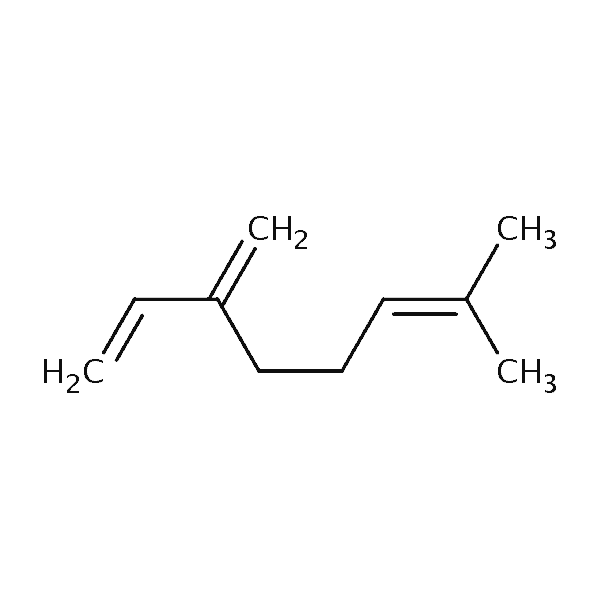Limonene 451 45 760 136Linalool 471 50 760 154Carophyllene 403 65.5 760 204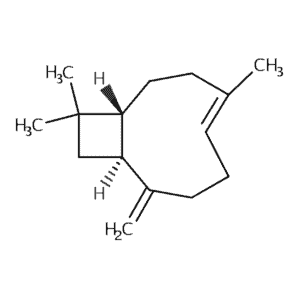Alpha-pinene 430 45 760 136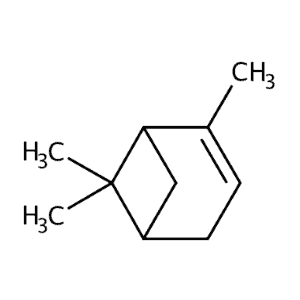Geraniol 503 63 750 154.2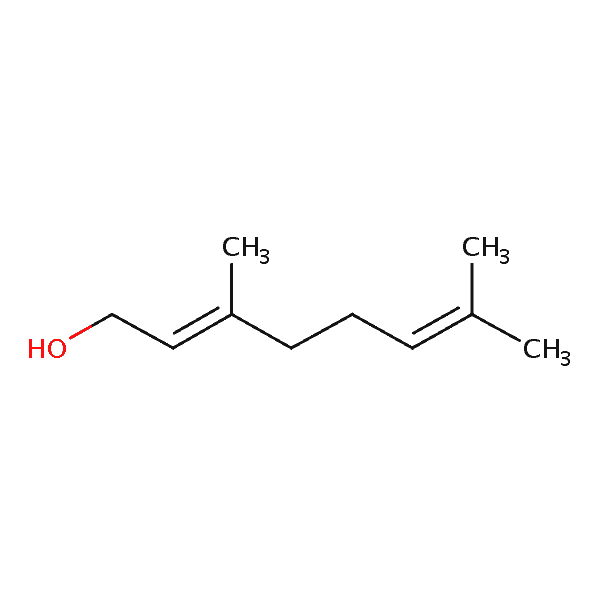Isoprene 307 26 750 68.1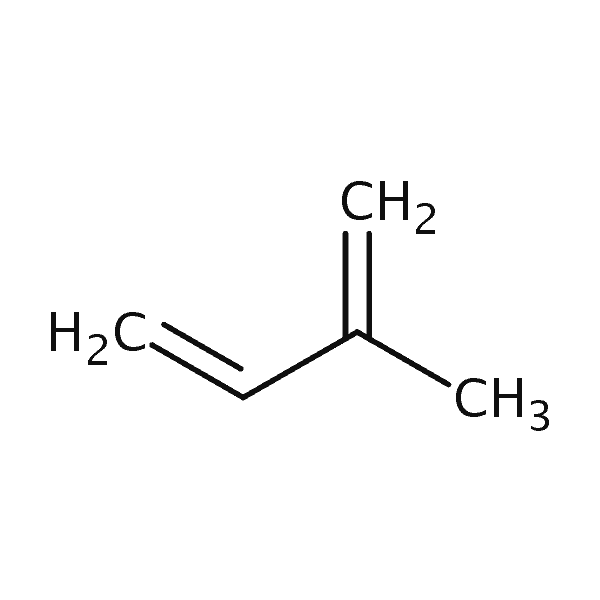p-Cymene 395 24 750 134.2Some key points that need to be discussed with this table include the following:

1. The enthalpies of vaporization are only validated for a small temperature range. The temperature ranges are given in the nist database and can be looked up easily.
2. If you prefer Celsius units, convert the boiling point given in degrees Kelvin to degrees C by subtracting 273.1. The calculator uses Kelvin units.
3. Notice that compounds with oxygen have higher enthalpy of vaporization.

## Use our Terpene Chart & Vacuum Boiling Point Calculator!

By taking the parameters in the above terpene chart, you can use our vacuum boiling point calculator using the Clausius Claperyon Equation. Click below to visit our calculator, adjust the values to represent the boiling point you want to calculate and input the pressure at which new boiling points are to be calculated!